# Tutorial 9: The Remaining Sentential Rules of Inference

Logical System

2013

### Skills to be acquired:

Learning the Rules Or Elimination and the Introduction of the Biconditional.

Bergmann The Logic Book Section 5.1 and 5.4

### The Tutorial:

Or Elimination, in the guise of Dilemma, also is a form of inference dating from antiquity.

The core idea of it that if a conclusion follows from both disjuncts of a disjunction, then the conclusion follows full stop. As an example in English, if either I am going to eat an ice-cream or I am going to eat some cake, and if I eat ice-cream I break my diet, and if I eat cake I break my diet, then ... I break my diet.

In our rule system dilemma appears as the rule for the Elimination of Disjunction ∨E. This, then, is a rule which gets used when you have a formula which has disjunction as its main connective and are trying to obtain some other formula.

The circumstance that Elimination of Disjunction gets invoked looks like this.That is, a formula you have has a ∨ as its main connective-- the formula you have has the form <left disjunct> ∨ <right disjunct>. In the illustration, for example, F is the <left disjunct> and G is the <right disjunct>. And there is a <target> which is the formula you are trying to obtain. In the illustration H is the <target>.

You follow a five stage process. First you assume the <left disjunct>. The illustrated fragment will then become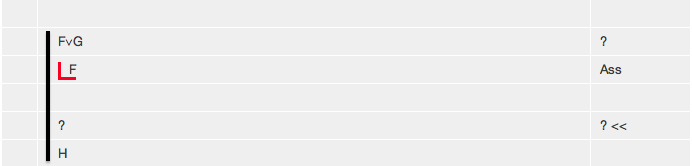Second you continue deriving in this new context until you manage to derive <target> thusThird you drop the assumption of the <left disjunct> and assume instead the <right disjunct>. You do this by clicking the Rule Assumption, entering the <right disjunct>. and choosing 'Drop Last' . The illustrated fragment will then become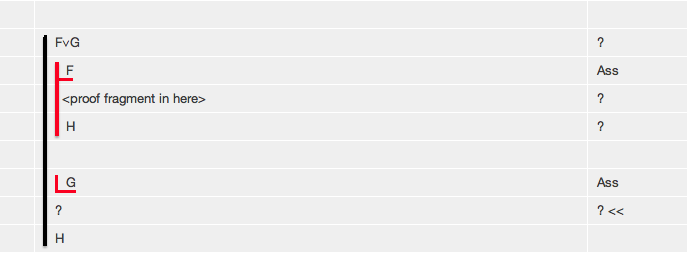Fourth, you continue deriving in this new context until you manage to derive <target> thus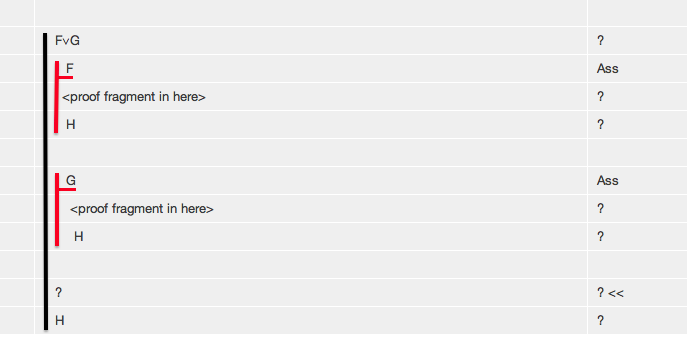Finally you select three items -- the disjunct formula, and the last lines of the two sub-proofs (each with the same <target> formula on them) and click ∨E to complete the Or elimination.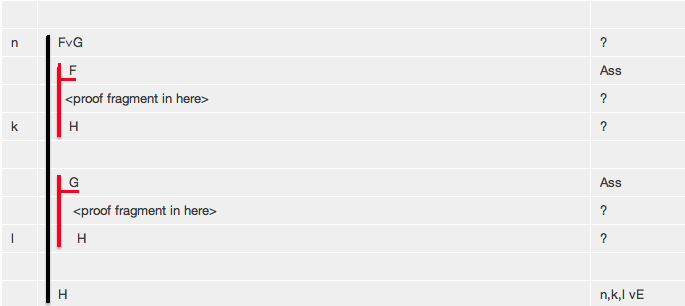The final propositional Rule--the Introduction of the Biconditional-- is similar to two conditional proofs, one going the left to right, and the other from right to left. It gets used when you are trying to obtain a biconditional formula.

The circumstance it gets invoked looks like this.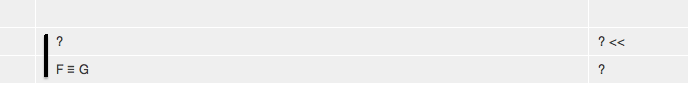That is, the formula you are trying to obtain has a ≡ as its main connective-- the formula you are trying to obtain has the form <left> ≡ <right>. In the illustration, for example, F is the <left> and G is the <right> .

You follow a five stage process. First you assume the <left>. The illustrated fragment will then becomeSecond you continue deriving in this new context until you manage to derive the <right> thusThird you drop the <left> assumption and instead assume the <right>. You do this by clicking the Rule Assumption, entering the <right>. and choosing 'Drop Last' . The illustrated fragment will then become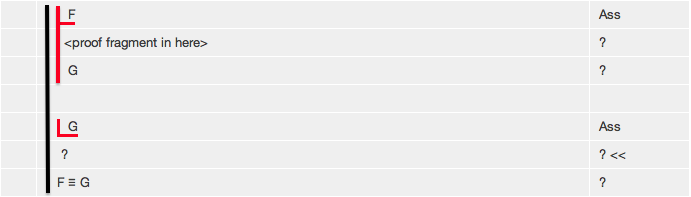Fourth you continue deriving in this new context until you manage to derive the <right> thus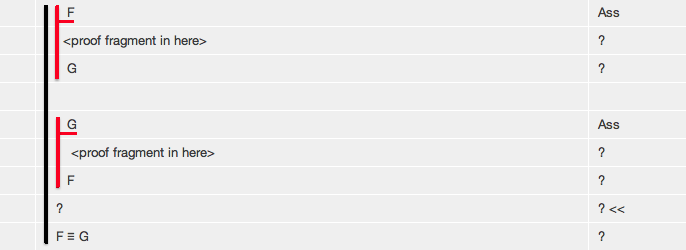Finally you select the last lines of the two subproofs (that is, <right> and <left>) and click ≡I to complete the Biconditional Introduction.## Exercises to accompany Tutorial 9

### Exercise 1 (of 5).

Derive the following using Tactics. (Start the derivation, switch Tactics on, select the disjunt formula, and click ∨E-- this will lay out the derivation for you.) Then repeat the derivations without using Tactics.

a) F∨G,F⊃H,G⊃H∴H
b) (F&F)∨(G&G),F⊃H,G⊃H∴H
c) F∨G ∴G∨F
d) (F⊃G)&(H⊃D), F∨H ∴ G∨D

### Exercise 2 (of 5). (*slightly more difficult*)

Derive the following.

a) F∨(G&∼G)∴ F
b) F∨G, G⊃H ∴ ∼F⊃H
c) ∼F&∼G∴ ∼(F∨G)

### Exercise 3 (of 5). (*slightly more difficult*)

There are a number of important theorems which can be expressed as equivalences-- here we consider slightly weakened versions of them which are conditionals.

Derive

De Morgan's Laws

a) ∴ (∼F&∼G)⊃∼(F∨G)

Distribution

b) ∴ (F&(G∨H))⊃((F&G)∨(F&H))

c) ∴ ((F∨G)&(F∨H))⊃(F∨(G&H))

d) ∴ (F&(G∨H))⊃((F&G)∨(F&H))

e) ∴ ((F∨G)&(F∨H))⊃(F∨(G&H))

Implication

f) ∴ (∼F∨G)⊃(F⊃G)

### Exercise 4 (of 5).

Derive the following with or without Tactics. (Start the derivation, switch Tactics on, and click ≡I --this will lay out the derivation for you.) Then do the derivations without using Tactics.

a) (F⊃G)&(G⊃F) ∴ F≡G
b) ∴ (F&F)≡F
c) ∴ (F∨F)≡F

### Exercise 5 (of 5).

There are a number of well-known theorems which express equivalences between formulas, derive them

Association

a) ∴ F∨(G∨H)≡(F∨G)∨H
b) ∴ F&(G&H)≡(F&G)&H

Commutation

c) ∴ F∨G≡G∨F
d) ∴ F&G≡G&F

De Morgan's Laws

e) ∴ ∼(F&G)≡∼F∨∼G
f) ∴ ∼(F∨G)≡∼F&∼G

Distribution

g) ∴ F&(G∨H)≡(F&G)∨(F&H)
h) ∴ F∨(G&H)≡(F∨G)&(F∨H)

Double Negation

i) ∴ F≡∼∼F

Equivalence

j) ∴ (F≡G)≡(F⊃G)&(G⊃F)
k) ∴ (F≡G)≡(F&G)∨(∼F&∼G)

Exportation

l) ∴ (F⊃(G⊃H))≡((F&G)⊃H)

Implication

m) ∴ F⊃G≡∼F∨G

Transposition

n) ∴ F⊃G≡∼G⊃∼F

If you decide to use the web application for the exercises you can launch it from here Deriver[Bergmann] — username 'logic' password 'logic'. Then either copy and paste the above formulas into the Journal or use the Deriver File Menu to Open Web Page with this address https://softoption.us/test/easyDeriver/CombinedExercisesEasyDBergmann.html .

Preferences

You may need to set some Preferences for this.

• you can check that the parser is set to bergmann.